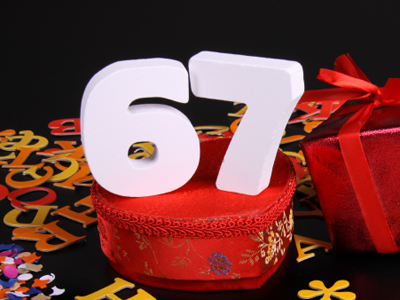The best method of multiplying 67 by 50 is to multiply by 100 then halve.

# Multiplication and Division (Year 5)

In KS2 Maths children will come to understand multiplication and division. In Year Five children should have a good grasp of their times tables and also be capable of doing some multiplication and division involving much larger numbers. They should also be confident when multiplying or dividing with simple fractions and decimals.

Multiplication and division involves splitting numbers into parts, or fractions (in the case of division). Some divisions are straightforward, such as dividing 20 bananas between 4 monkeys. Each monkey will get 5 bananas each. Other divisions are not so simple! Multiplying and dividing can be quite hard with decimals and fractions, but once you know how to go about it it does get easier! To multiply a number by 14 you divide it by 4. The same is true for decimals: to multiply a number by 0.25 you also divide it by 4.

Test your knowledge of multiplication and division by trying the following quiz on the subject.

1.
What is the best method of multiplying 58 by 9?
Multiply by 10 then add 58
Multiply by 5 then add 58
Multiply by 10 then subtract 58
Multiply by 5 then subtract 58
10 is a much easier number to multiply than 9 is
2.
3 + (5 x 8) =
(3 + 5) x 8 =
3 + 5 x 8 =
(3 + 8) x 5 =
The calculation in the brackets is always done first
3.
How can one twentieth be calculated?
Find one tenth and halve
Find one fifth and double
Find one tenth and double
Find one fifth and halve
One twentieth is half of one tenth
4.
On a calculator the answer to a division is 7.2. How is this interpreted in money?
£72
£7.2
£7.20
£0.72
720p is the same as £7.20
5.
Which of these methods would result in finding 16?
Half one third
Half one quarter
Double one quarter
Double one third
A sixth is half a third
6.
Which strategy would be best to multiply 79 by 21?
Multiply by 10 then subtract 79
Multiply by 20 then add 79
Multiply by 10 then add 79
Multiply by 20 then subtract 79
To simplify it further you could multiply 79 by 2, then by 10 and finally add 79
7.
22 ÷ 4 = 5 r 2. What fraction is the remainder 2?
Half
Quarter
Third
Eighth
The remainder can be expressed as 24 or 12
8.
How do we find a third of a number?
Multiply it by 3
Divide it by 6
Multiply it by 6
Divide it by 3
A third means the same as ÷ 3
9.
Which strategy would be best to multiply 42 by 16?
Multiply by 8 then double
Multiply by 4 then double
Divide by 2 then multiply by 8
Divide by 8 then double
If a number in a problem is difficult to work with then try to break it down into easier to deal with numbers
10.
What is the best method of multiplying 67 by 50?
Multiply by 100 then halve
Multiply by 100 then double
Multiply by 5 then multiply by 10
Divide by 100 then double
100 and 2 are easier numbers to multiply and divide than 50 is